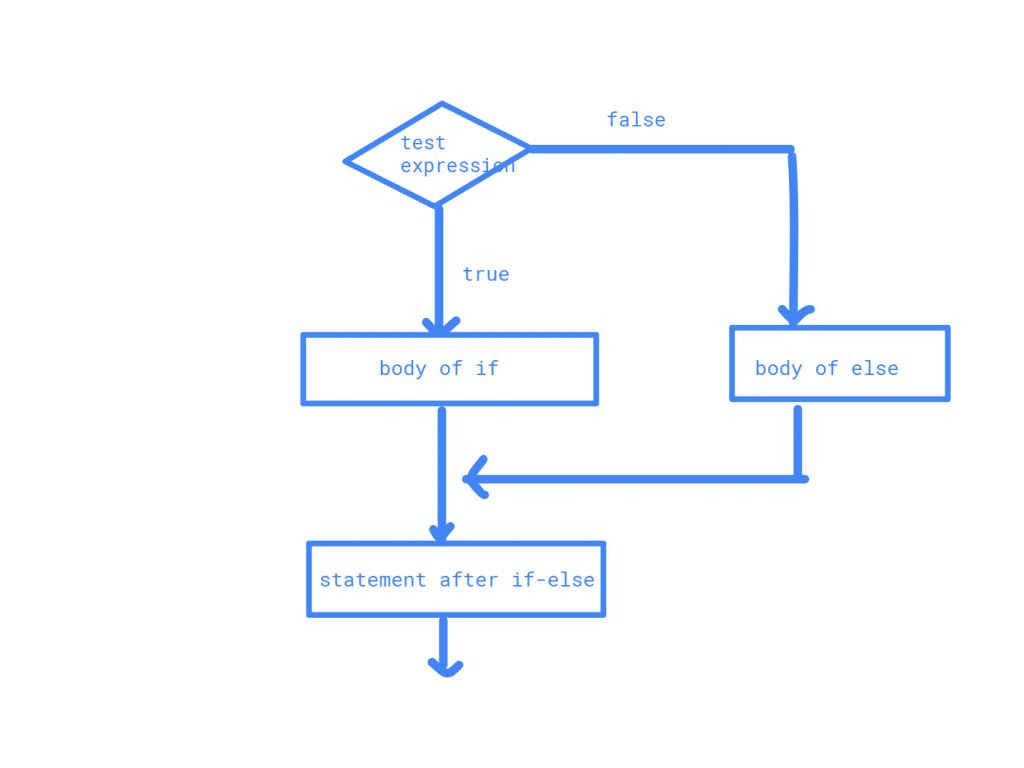# If- Else Statements in JavaIt is a conditional statement, with which you may take one or more test conditions.there are 3 types

• If statement
• If-else statement
• nested if-else

### 1.if statement#

only if statement has only one condition ,if the condition fails it skips to next statement.

syntax

if(condition){
Statement(s);
}

flowchartExample Program

import java.util.*;
class iffun
{
public static void main(String args[])
{
Scanner s=new Scanner(System.in);
int x=s.nextInt();
if(x>18)
{
System.out.println("you are allowed to enter this website");
}
s.close();
}
}

Output

20
you are allowed to enter this website

### 2.If-else Statement#

if -else is used for both true and false for a condition.

syntax

if (expression)
sattement1;
else
statement2;

flowcharthere, if the condition is true .next statement1 will be executed and skips statement2

if the condition is false next statement2 will be executed and skips satement1. using else part is not mandatory, let’s see one example

Example Program

import java.util.*;
class ifelsefun
{
public static void main(String args[])
{
Scanner s=new Scanner(System.in);
int x=s.nextInt();
if(x>18)
{
System.out.println("you are allowed to enter this website");
}
else{
System.out.println("you are not allowed to enter this website");
}
s.close();
}
}

Output

14
you are not allowed to enter this website

here, in this example, the condition is, the age of the person should be either 18 or more to enter the website. that means if the test expression given (a>=18) is true,it displays the first message”you are allowed to enter this website”,it it is false, then the second message is displayed “you are not allowed to enter this website”.

### 3. If else-if ladder Statement#

if else-if statement is used to work on multiple conditions .if the respective conditions are true then their statements are executed, if they are false it skips to next condition

syntax

if(condition1){
//code to be executed if condition1 is true
}
else if(condition2){
//code to be executed if condition2 is true
}
else if(condition3){
//code to be executed if condition3 is true
}
Else{
//code to be executed if all the conditions are false
}

flowchart

import java.util.*;
class ifelseif
{
public static void main(String args[])
{
Scanner s=new Scanner(System.in);
System. out. println("Enter x 1 or 2 or 3");
int x=s.nextInt();
if(x==1){
System.out.println("x is equals to 1");
}
else if(x==2){
System.out.println("x is equal to 2");
}
else if(x==3){
System.out.println("x is equal to 3");
}
else{
System.out.println("x is not equal to 1, 2 or 3");
}
s.close();
}
}

Output

Enter x 1 or 2 or 3
2
x is equal to 2

### 4.nested if-else#

writing if-else in another if-else is called nested if-else

syntax

if(condition1) {
//Nested if else inside the body of "if"
if(condition2) {
//Statements inside the body of nested "if"
}
else {
//Statements inside the body of nested "else"
}
}
else {
//Statements inside the body of "else"
}

until the condition 1 is true condition2 executes again and again ,when it is false else will be executed

example

import java.util.*;
class nestedif
{
public static void main(String args[])
{
Scanner s=new Scanner(System.in);
System.out.printf("Enter 3 numbers");
System.out.println(s.nextLine());
int n1=s.nextInt();
int n2=s.nextInt();
int n3=s.nextInt();
if (n1>=n2)
{
if(n1>=n3)
System.out.printf("%d is the largest number.", n1);
else
System.out.printf("%d is the largest number.", n3);
}
else
{
if(n2>=n3)
System.out.printf("%d is the largest number.", n2);
else
System.out.printf("%d is the largest number.",n3);
}
s.close();
}
}

output

Enter 3 numbers
46
43
17
46 is the largest number.
Last updated on by vishal devxo